Optimizing RNNs with Differentiable Graphs
 Jesse Engel Baidu Silicon Valley AI Lab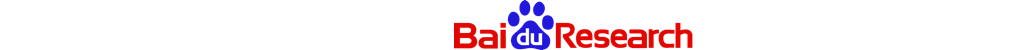## 1. Preamble

This is Part II of a multi-part series detailing some of the techniques we've used here at Baidu's Silicon Valley AI Lab (SVAIL) to accelerate the training of recurrent neural networks. While Part I focused on the role that minibatch and memory layout play on recurrent GEMM performance, we shift our focus here to tricks that we can use to optimize the algorithms themselves.

## 2. Summary

The main takeaways of this blog post are:

1. Differentiable graphs are a simple and useful tool for visually calculating complicated derivatives.

2. These graphs can also inspire algorithmic optimizations. As an example, we show how to accelerate Gated Recurrent Units (GRUs) by up to 40%.

• Applying the reset gate after the recurrent multiply contributes ~10% of the speedup without loss in accuracy.

### 2.1. Audience

This post will hopefully be helpful for:

1. Researchers using frameworks that require explicit gradient calculation, such as Torch or Caffe. We will see how to easily visualize and infer gradients in terms of GPU kernels.

2. Researchers developing new iterative algorithms. We will develop variations of iterative algorithms such as RNNs that are more efficiently parallelized.

3. The authors of Deep Learning frameworks that apply auto-differentiation such as Theano, Tensorflow, Torch Autograd, or Neon. These methods will hopefully provide inspiration for implicit graph optimizations to move towards systems that can better balance tradeoffs of memory usage and computation.

## 3. Introduction

One of the main reasons for the recent success and popularity of deep learning algorithms is that their performance scales well with dataset size. For example, in our recent work on Deep Speech 2, we found that the word error rate (WER) of our speech system decreases by 40% for every decade increase in data. We see that this power law trend holds over two orders of magnitude of data.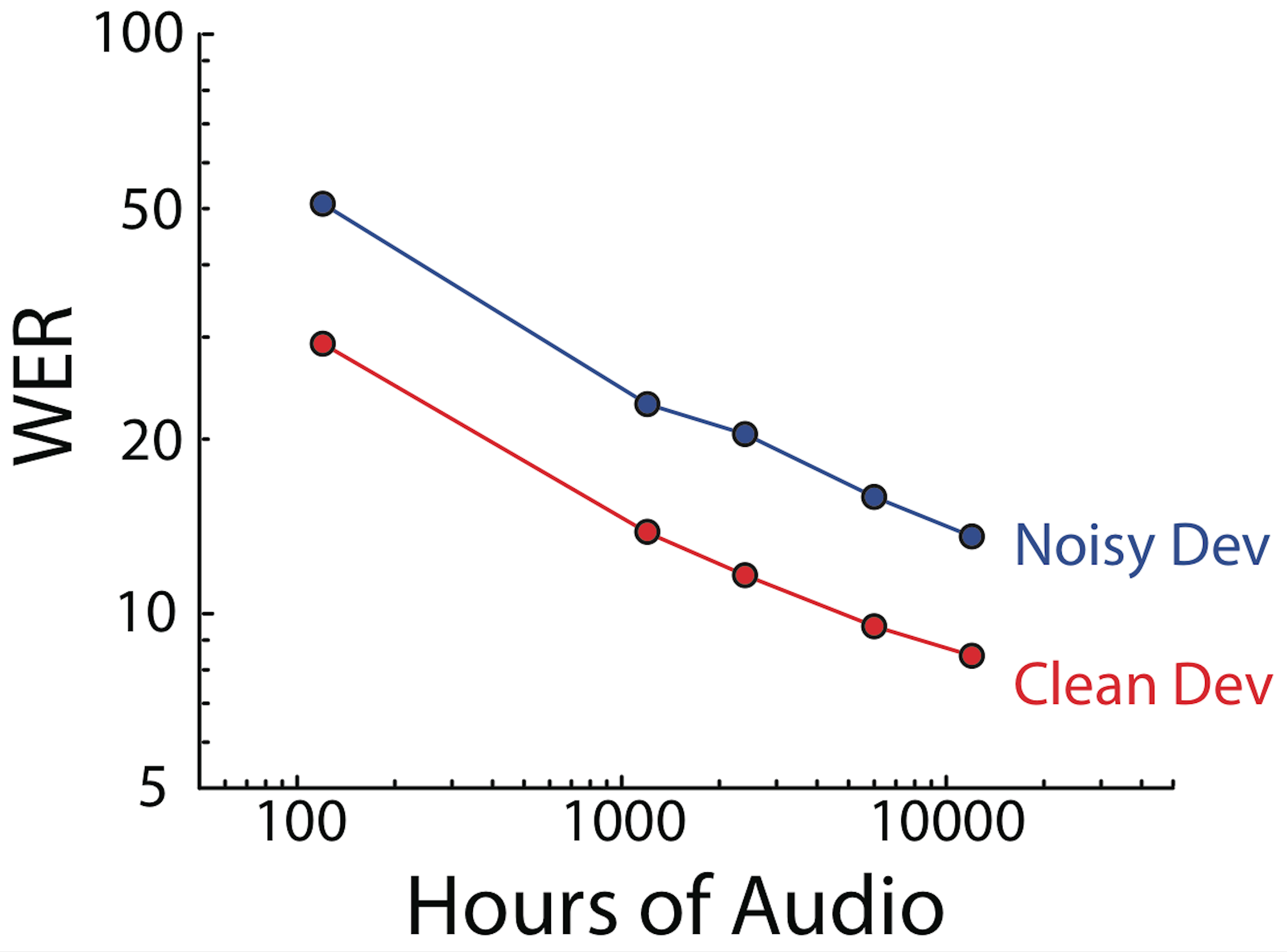Good performance is thus closely tied with being able to train quickly on increasing amounts of data. Deep learning's success is partly due to its core operations, such as matrix multiplication and convolution, having very efficient GPU kernel implementations (e.g. cuBLAS and cuDNN respectively).

Recurrent Neural Networks (RNNs) have iterative dependencies that make them well-suited for sequential tasks, but tricky to efficiently parallelize. Check out Part I of this series for an in-depth review of efficient size/minibatch design principles for RNNs. As with other “neural” operations, the most efficient RNNs require custom GPU implementations such as in cuDNNv5 or in our Persistent RNN kernels.

Imagine, however, that you are developing a new algorithm that doesn't yet have a custom implementaiton (part of the joy of machine learning research). In this blog post, using RNNs as a case study, we want to explore the question:

What optimizations can we perform when composing a new algorithm out of existing kernels?

## 4. Differentiable Graph Notation

We will assume the reader has basic familiarity with the backpropagation algorithm. While we avoid derivations in the current discussion for brevity, we highly encourage interested readers to read the derivations in the appendix.

We consider a computational graph composed of nested operations, that turn input tensors, , into output tensors, . If these tensors are not parameters of the model, we call them “activations”. The derivative of a cost function, , with respect to any tensor in the graph, , can be decomposed into an “error” term, , and a derivative of the operation . On backprop, the error term propagates from operation to operation, accumulating the derivative of each operation along the way. Thus, becomes for the next operation.

(1)

As an electrical engineer in a previous life, I'm biased to represent the activations as the edges of the graph (like voltages) and the functions as the nodes (like circuit elements). If we also express parameters as nodes, then all of our functions are stateless and the graph is explicit. I find that grounding the visualization in the physics of circuits to be helpful and is similar to approaches by Chris Olah and Andrej Karpathy.1

Let's look at the basic operations we'll use in most differentiable algorithms. Many more complicated operations can be decomposed into these fundamental kernels: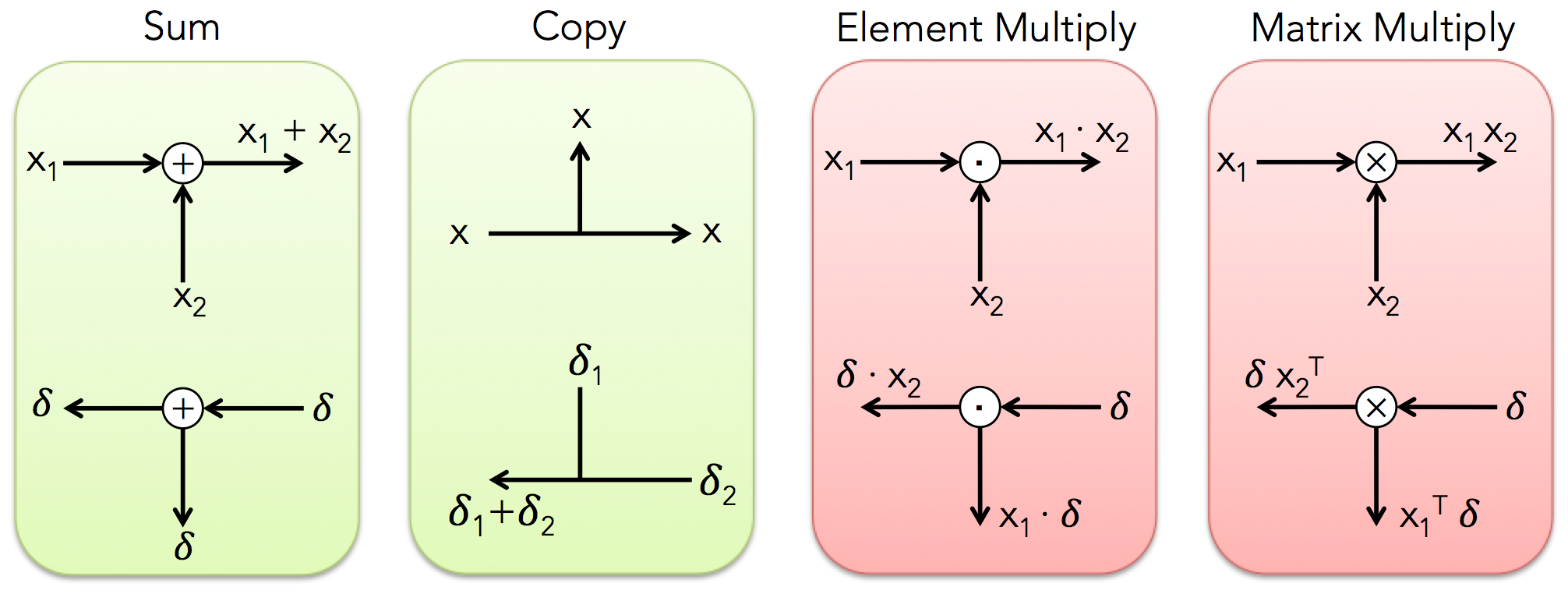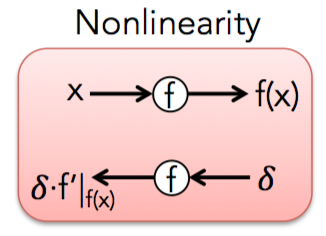where the top diagram is the forward prop rule (up and to the right), and the bottom is the backprop rule (down and to the left). Some things to note:

• Sum and copy operators are complimentary. Applying one on forward prop is equivalent to apply the other on backprop.

• The copy operator is implicit in the splitting of the wire as it's intuitive from the circuit point of view (a connected wire shares the same voltage everywhere).

• For multiplication operators on backprop, the error propagates down each input and is multiplied by the activations from the other input (ex. ).

• For matrix multiplication, do the same, except also take the transpose of the activations and preserve the original order so that the sizes match (ex. ). 2

• It is often convenient to evaluate the derivative of nonlinearities, , at the postactivation values, . See more in the appendix.

I've marked in red the operations where backprop also requires incoming activations. These are the operations for which we will need to decide whether to save or recompute these activations.

#### 4.0.1. Example: Fully Connected Layer

Before we dive into optimizing RNNs, let's take a look at the simple example of a fully connected layer

(2)

where is an elementwise nonlinearity, and and are weight and bias parameters respectively. We can thus draw the forward pass as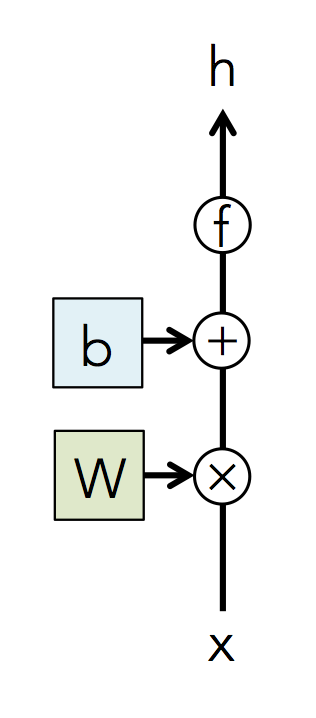and then use our graph propagation rules to read out the derivatives from the backward pass.